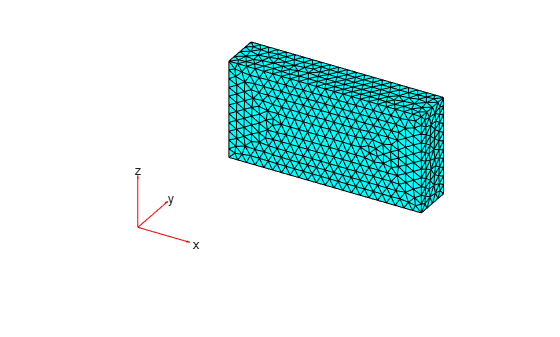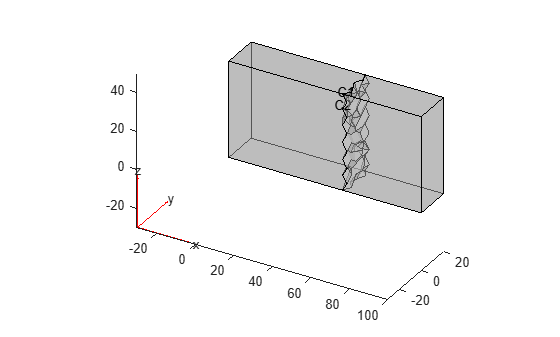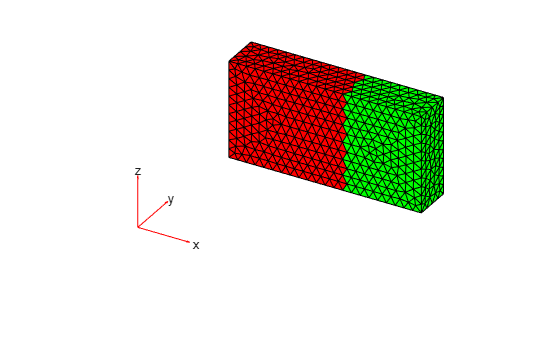Main Content

# Multidomain Geometry Reconstructed from Mesh

This example shows how to split a single-domain block geometry into two domains. The first part of the example generates a mesh and divides the mesh elements into two groups. The second part of the example creates a two-domain geometry based on this division.

### Generate Mesh and Split Its Elements into Two Groups

Create a PDE model.

`modelSingleDomain = createpde;`

Import the geometry.

`importGeometry(modelSingleDomain,'Block.stl');`

Generate and plot a mesh.

```msh = generateMesh(modelSingleDomain); figure pdemesh(modelSingleDomain)```Obtain the nodes and elements of the mesh.

```nodes = msh.Nodes; elements = msh.Elements;```

Find the x-coordinates of the geometric centers of all elements of the mesh. First, create an array of the same size as `elements` that contains the x-coordinates of the nodes forming the mesh elements. Each column of this vector contains the x-coordinates of 10 nodes that form an element.

`elemXCoords = reshape(nodes(1,elements),10,[]);`

Compute the mean of each column of this array to get a vector of the x-coordinates of the element geometric centers.

`elemXCoordsGeometricCenter = mean(elemXCoords);`

Assume that all elements have the same region ID and create a matrix `ElementIdToRegionId`.

`ElementIdToRegionId = ones(1,size(elements,2));`

Find IDs of all elements for which the x-coordinate of the geometric center exceeds 30.

`idx = mean(elemXCoords) > 30;`

For the elements with centers located beyond x = 30, change the region IDs to 2.

`ElementIdToRegionId(idx) = 2;`

### Create Geometry with Two Cells

Create a new PDE model.

`modelTwoDomain = createpde;`

Using `geometryFromMesh`, import the mesh. Assign the elements to two cells based on their IDs.

`geometryFromMesh(modelTwoDomain,nodes,elements,ElementIdToRegionId)`
```ans = DiscreteGeometry with properties: NumCells: 2 NumFaces: 108 NumEdges: 205 NumVertices: 100 Vertices: [100x3 double] ```

Plot the geometry, displaying the cell labels.

`pdegplot(modelTwoDomain,'CellLabels','on','FaceAlpha',0.5)`Highlight the elements from cell 1 in red and the elements from cell 2 in green.

```elementIDsCell1 = findElements(modelTwoDomain.Mesh,'region','Cell',1); elementIDsCell2 = findElements(modelTwoDomain.Mesh,'region','Cell',2); figure pdemesh(modelTwoDomain.Mesh.Nodes, ... modelTwoDomain.Mesh.Elements(:,elementIDsCell1), ... 'FaceColor','red') hold on pdemesh(modelTwoDomain.Mesh.Nodes, ... modelTwoDomain.Mesh.Elements(:,elementIDsCell2), ... 'FaceColor','green')```## SupportGet trial now